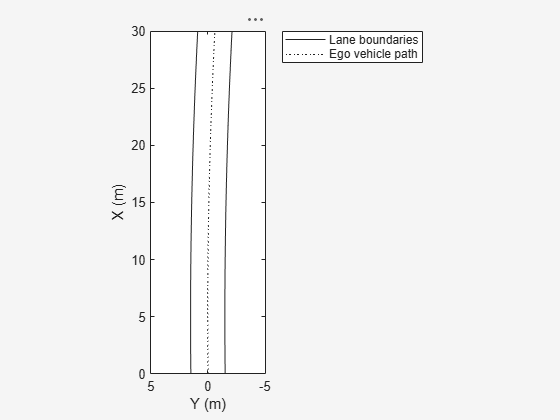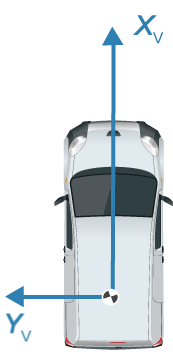Main Content

# plotPath

Display actor paths on bird’s-eye plot

## Syntax

``plotPath(pPlotter,pathCoords)``

## Description

example

````plotPath(pPlotter,pathCoords)` displays the paths of actors from a list of path coordinates on a bird's-eye plot. The path plotter object, `pPlotter`, is associated with a `birdsEyePlot` object and configures the display of the specified path.To remove all paths associated with the path plotter `pPlotter`, call the `clearData` function and specify `pPlotter` as the input argument.```

## Examples

collapse all

Create a 3-meter-wide lane.

```lb = parabolicLaneBoundary([-0.001,0.01,1.5]); rb = parabolicLaneBoundary([-0.001,0.01,-1.5]);```

Compute the lane boundary model manually from 0 to 30 meters along the x-axis.

```xWorld = (0:30)'; yLeft = computeBoundaryModel(lb,xWorld); yRight = computeBoundaryModel(rb,xWorld);```

Create a bird's-eye plot and lane boundary plotter. Display the lane information on the bird's-eye plot.

```bep = birdsEyePlot('XLimits',[0 30],'YLimits',[-5 5]); lanePlotter = laneBoundaryPlotter(bep,'DisplayName','Lane boundaries'); plotLaneBoundary(lanePlotter,{[xWorld,yLeft],[xWorld,yRight]});```Create a path plotter. Create and display the path of an ego vehicle that travels through the center of the lane.

```yCenter = (yLeft + yRight)/2; egoPathPlotter = pathPlotter(bep,'DisplayName','Ego vehicle path'); plotPath(egoPathPlotter,{[xWorld,yCenter]});```## Input Arguments

collapse all

Path plotter, specified as a `PathPlotter` object. This object is stored in the `Plotters` property of a `birdsEyePlot` object and configures the display of the specified actor paths in the bird's-eye plot. To create this object, use the `pathPlotter` function.

Path coordinates, specified as a cell array of M-by-2 real-valued matrices. Each matrix represents the coordinates for a different path. M is the number of coordinates in a path and can be different for each path. The first and second columns of each matrix represent the (X, Y) positions of the path curve. The positive X-direction points ahead of the center of the vehicle. The positive Y-direction points to the left of the origin of the vehicle, which is the center of the rear axle, as shown in this figure of the vehicle coordinate system..Path coordinates are relative to the ego vehicle.

## See Also

Introduced in R2017a

## Support

#### Implementing an Adaptive Cruise Controller with Simulink

Download technical paper